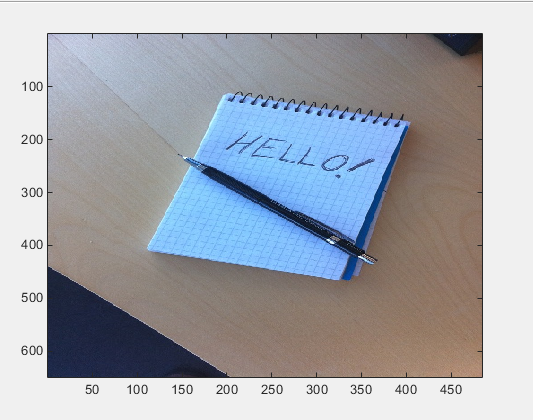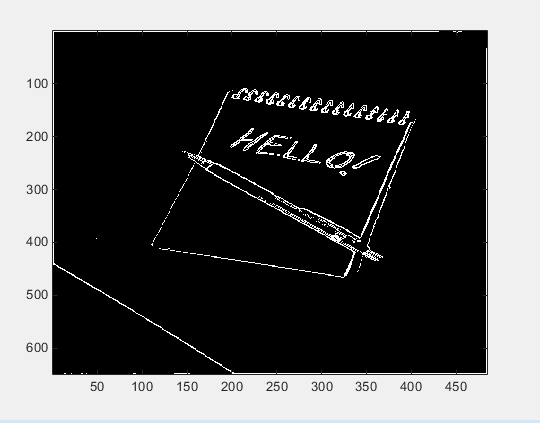## 在应用程序中使用示例 C 主函数

### 前提条件

• MATLAB

• MATLAB Coder™

• C 编译器（对于大多数平台，MATLAB 随附提供了一个默认 C 编译器）。要查看支持的编译器列表，请参阅支持的编译器

可以使用 `mex -setup` 更改默认编译器。请参阅更改默认编译器

### 创建文件夹并复制相关文件

`sobel.m`函数代码Sobel 滤波算法的 MATLAB 实现。`sobel.m` 接受图像（表示为双精度矩阵）和阈值作为输入。该算法检测图像中的边缘（基于阈值）。`sobel.m` 返回修改后且显示边缘的图像。
`hello.jpg`图像文件Sobel 滤波器修改的图像。

1. 创建一个本地工作文件夹。例如，`c:\coder\edge_detection`

2. 导航到该工作文件夹。

3. 将文件 `sobel.m``hello.jpg` 从示例文件夹 `sobel` 复制到工作文件夹。

`copyfile(fullfile(docroot, 'toolbox', 'coder', 'examples', 'sobel'))`

### 对图像运行 Sobel 滤波器

1. 将原始图像读入 MATLAB 矩阵并显示该图像。

`im = imread('hello.jpg');`
2. 显示该图像以便与 Sobel 滤波器结果进行比较。

`image(im);`3. Sobel 滤波算法对灰度图像进行运算。使用归一化值（0.0 代表黑色、1.0 代表白色），将彩色图像转换为等效的灰度图像。

`gray = (0.2989 * double(im(:,:,1)) + 0.5870 * double(im(:,:,2)) + 0.1140 * double(im(:,:,3)))/255;`
4. 要运行 Sobel 滤波器的 MATLAB 函数，请将灰度图像矩阵 `gray` 和阈值传递给函数 `sobel`。此示例使用 0.7 作为阈值。

`edgeIm = sobel(gray, 0.7);`
5. 要显示修改后的图像，请使用 `repmat` 函数重新格式化矩阵 `edgeIm`，以便将其传递给 `image` 命令。

```im3 = repmat(edgeIm, [1 1 3]); image(im3);```### 生成并测试 MEX 函数

1. 要测试生成的代码是否在功能上等效于原始 MATLAB 代码并且不会发生运行时错误，请生成一个 MEX 函数。

`codegen -report sobel`

`codegen` 在当前工作文件夹中生成名为 `sobel_mex` 的 MEX 函数。

2. 要运行 Sobel 滤波器的 MEX 函数，请将灰度图像矩阵 `gray` 和阈值传递给函数 `sobel_mex`。此示例使用 0.7 作为阈值。

`edgeImMex = sobel_mex(gray, 0.7);`
3. 要显示修改后的图像，请使用 `repmat` 函数重新格式化矩阵 `edgeImMex`，以便将其传递给 `image` 命令。

```im3Mex = repmat(edgeImMex, [1 1 3]); image(im3Mex);```

此图像与使用 MATLAB 函数创建的图像相同。

### 为 sobel.m 生成示例主函数

1. 为 C 静态库创建一个配置对象。

`cfg = coder.config('lib');`

对于 C/C++ 源代码、静态库、动态库和可执行文件的配置对象，设置 `GenerateExampleMain` 可控制示例主函数的生成。默认情况下，该设置设为 `'GenerateCodeOnly'`，即生成示例主函数但不编译它。对于此示例，请勿更改 `GenerateExampleMain` 设置的值。

2. 使用该配置对象生成一个 C 静态库。

`codegen -report -config cfg sobel`

### 修改生成的示例主函数

C 主函数必须满足您的应用程序的要求。此示例修改示例主函数以满足 Sobel 滤波器应用程序的要求。

• 从二进制文件中读入灰度图像。

• 应用 Sobel 滤波算法。

• 将修改后的图像保存为二进制文件。

#### 修改 main 函数

`main` 函数修改为：

• 接受包含灰度图像数据的文件和阈值作为输入参数。

• 使用灰度图像数据流的地址和阈值作为输入参数调用函数 `main_sobel`

1. 删除 `void(argc)``(void)argv` 声明。

2. 声明变量 `filename` 以保存包含灰度图像数据的二进制文件的名称。

`const char *filename;`
3. 声明变量 `threshold` 以保存阈值。

`double threshold;`
4. 声明变量 `fd` 以保存应用程序从 `filename` 读入的灰度图像数据的地址。

`FILE *fd;`
5. 添加一个用于检查三个参数的 `if` 语句。

```if (argc != 3) { printf("Expected 2 arguments: filename and threshold\n"); exit(-1); }```
6. 将包含灰度图像数据的文件的输入参数 `argv` 赋给 `filename`

`filename = argv;`
7. 将阈值的输入参数 `argv` 赋给 `threshold`，从而将输入从字符串转换为双精度数值。

`threshold = atof(argv);`
8. 打开包含其名称在 `filename` 中指定的灰度图像数据的文件。将数据流的地址赋给 `fd`

`fd = fopen(filename, "rb");`
9. 要验证可执行文件可以打开 `filename`，请编写一个 `if` 语句，其功能是在 `fd` 的值为 `NULL` 时退出程序。

```if (fd == NULL) { exit(-1); }```
10. 将对 `main_sobel` 的函数调用替换为带输入参数 `fd``threshold` 调用 `main_sobel`

`main_sobel(fd, threshold);`
11. 调用 `sobel_terminate` 后关闭灰度图像文件。

`fclose(fd);`

#### 修改初始化函数 argInit_d1024xd1024_real_T

1. 将输入参数 `void` 替换为参数 `FILE *fd`。此变量指向该函数读入的灰度图像数据。

`static emxArray_real_T *argInit_d1024xd1024_real_T(FILE *fd)`
2. 更改变量 `iv2` 的值以匹配灰度图像矩阵 `gray` 的维度。`iv2` 保存 `argInit_d1024xd1024_real_T` 创建的 emxArray 维度的大小值。

`static int iv2 = { 484, 648 };`

MATLAB 以列优先格式存储矩阵数据，而 C 以行优先格式存储矩阵数据。请相应地声明维度。

3. 定义变量 `element` 以保存从灰度图像数据读入的值。

`double element;`
4. 通过向内部 `for` 循环添加 `fread` 命令，更改 `for` 循环构造以将数据点从归一化图像读入 `element` 中。

`fread(&element, 1, sizeof(element), fd);`
5. `for` 循环内，将 `element` 指定为 emxArray 数据的值集合。

`result->data[b_j0 + result->size * b_j1] = element;`

#### 编写 saveImage 函数

MATLAB 函数 `sobel.m` 与 MATLAB 数组交互，而 Sobel 滤波器应用程序与二进制文件交互。

1. 定义函数 `saveImage`，它将 emxArray `edgeImage` 的地址作为输入，输出类型为 void。

```static void saveImage(emxArray_uint8_T *edgeImage) { }```
2. 定义变量 `b_j0``b_j1`，就像在函数 `argInit_d1024xd1024_real_T` 中定义它们一样。

```int b_j0; int b_j1;```
3. 定义变量 `element` 以存储从 emxArray 读取的数据。

`uint8_T element;`
4. 打开二进制文件 `edge.bin` 以写入修改后的图像。将 `edge.bin` 的地址赋给 `FILE *fd`

`FILE *fd = fopen("edge.bin", "wb");`
5. 要验证可执行文件可以打开 `edge.bin`，请编写一个 `if` 语句，其功能是在 `fd` 的值为 `NULL` 时退出程序。

```if (fd == NULL) { exit(-1); }```
6. 编写一个嵌套的 `for` 循环构造，该构造类似于函数 `argInit_d1024xd1024_real_T` 中的构造。

```for (b_j0 = 0; b_j0 < edgeImage->size[0U]; b_j0++) { for (b_j1 = 0; b_j1 < edgeImage->size[1U]; b_j1++) { } }```
7. 在内部 `for` 循环内，将来自修改后的图像数据的值赋给 `element`

`element = edgeImage->data[b_j0 + edgeImage->size * b_j1];`
8. 在为 `element` 赋值后，将来自 `element` 的值写入文件 `edge.bin`

`fwrite(&element, 1, sizeof(element), fd);`
9. `for` 循环构造后，关闭 `fd`

`fclose(fd);`

#### 修改 main_sobel 函数

• 将灰度图像数据的地址和阈值作为输入。

• 使用 `argInit_d1024xd1024_real_T` 从地址读取数据。

• 使用阈值 `threshold` 将数据传递给 Sobel 滤波算法。

• 使用 `saveImage` 保存结果。

1. 将函数的输入参数替换为 `FILE *fd``double threshold` 参数。

`static void main_sobel(FILE *fd, double threshold)`
2. 将输入参数 `fd` 传递给 `argInit_d1024xd1024_real_T` 的函数调用。

`originalImage = argInit_d1024xd1024_real_T(fd);`
3. 将对 `sobel` 的函数调用中的阈值输入替换为 `threshold`

`sobel(originalImage, threshold, edgeImage);`
4. 调用函数 `sobel` 后，使用输入 `edgeImage` 调用函数 `saveImage`

`saveImage(edgeImage);`

#### 修改函数声明

1. 将函数 `*argInit_d1024xd1024_real_T` 的输入更改为 `FILE *fd`

`static emxArray_real_T *argInit_d1024xd1024_real_T(FILE *fd);`
2. 将函数 `main_sobel` 的输入更改为 `FILE *fd``double threshold`

`static void main_sobel(FILE *fd, double threshold);`
3. 添加函数 `saveImage`

`static void saveImage(emxArray_uint8_T *edgeImage);`

#### 修改 include 文件

`#include <stdio.h>`

### 生成 Sobel 滤波器应用程序

1. 导航到工作文件夹（如果当前不在该文件夹中）。

2. 为 C 独立可执行文件创建一个配置对象。

`cfg = coder.config('exe');`
3. 使用该配置对象和修改后的主函数为 Sobel 滤波器生成 C 独立可执行文件。

`codegen -report -config cfg sobel main.c main.h`

### 运行 Sobel 滤波器应用程序

1. 创建 MATLAB 矩阵 `gray`（如果它当前不在 MATLAB 工作区中）：

`im = imread('hello.jpg');`
`gray = (0.2989 * double(im(:,:,1)) + 0.5870 * double(im(:,:,2)) + 0.1140 * double(im(:,:,3)))/255;`
2. 使用 `fopen``fwrite` 命令将矩阵 `gray` 写入一个二进制文件。应用程序读入此二进制文件。

```fid = fopen('gray.bin', 'w'); fwrite(fid, gray, 'double'); fclose(fid);```
3. 运行可执行文件，从而将文件 `gray.bin` 和阈值 0.7 传递给它。

要在 Windows 平台上运行 MATLAB 中的示例，请执行以下命令：

`system('sobel.exe gray.bin 0.7');`

可执行文件会生成 `edge.bin` 文件。

### 显示生成的图像

1. 使用 `fopen``fread` 命令将文件 `edge.bin` 读入 MATLAB 矩阵 `edgeImExe`

```fd = fopen('edge.bin', 'r'); edgeImExe = fread(fd, size(gray), 'uint8'); fclose(fd);```
2. 将矩阵 `edgeImExe` 传递给函数 `repmat` 并显示图像。

```im3Exe = repmat(edgeImExe, [1 1 3]); image(im3Exe);```

图像与来自 MATLAB 和 MEX 函数的图像匹配。

## 支持